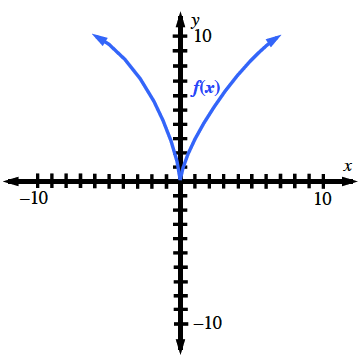### Home > APCALC > Chapter 5 > Lesson 5.1.3 > Problem5-30

5-30.

Mr. Lyon is trying to locate the points of inflection for $f\left(x\right) = 3x^{2/3}$. Since no value of $x$ exists such that $f^{\prime \prime}\left(x\right) = 0$, he assumes there is no change in concavity. Andrew says that a point of inflection exists where the second derivative changes sign. Who is correct and why? Use the second derivative and the graph of $y = f\left(x\right)$ to verify your answer.

CANDIDATES for points of inflection can be found where $f^{\prime \prime}\left(x\right) = 0$ and where $f^{\prime \prime}\left(x_{ }\right)$ DNE. You must check both.Notice that there is not a concavity change at the cusp.